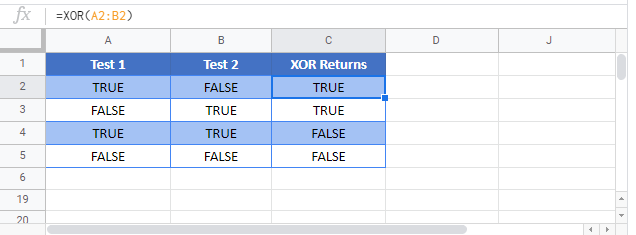XOR Function Examples – Excel, VBA, & Google Sheets

This tutorial demonstrates how to use the Excel XOR Function in Excel to test if one and only one criteria is true.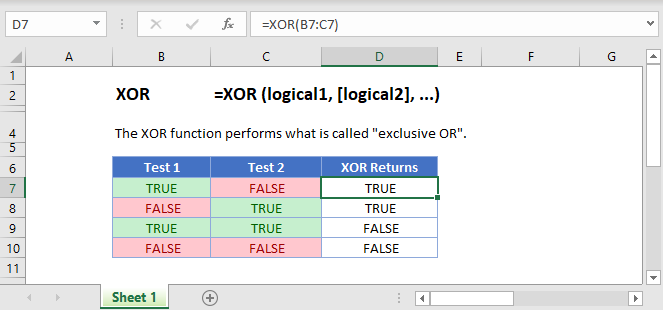XOR Function Description:

The XOR Function Checks whether one and only one condition is met. Returns TRUE or FALSE. If no conditions are met, or more than one condition is met, XOR returns FALSE. If only one condition is met, XOR returns TRUE.

To use the XOR Excel Worksheet Function, select a cell and type: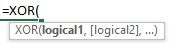(Notice how the formula inputs appear)

XOR function Syntax and inputs:

logical1 – Logical expressions. Example: A1 > 4.

What is the XOR Function?

XOR is one of Excel’s logical functions. It calculates something called an “exclusive OR” test (use the Excel OR Function<<link>> if you need to use an inclusive OR).

What is an Exclusive OR?

The Exclusive OR is a little more complex than the standard OR. It takes a series of arguments, and evaluates them as either TRUE or FALSE. Then:

• If an odd number of arguments are TRUE, XOR returns TRUE
• If an even number of arguments are TRUE, XOR returns FALSE
• If no arguments are TRUE, XOR also returns FALSE (zero is an even number)

How to Use the XOR Function

With the Excel XOR Function, you supply a number of arguments. XOR first evaluates these arguments as TRUE or FALSE, and then performs the exclusive OR test on the results. See the example below: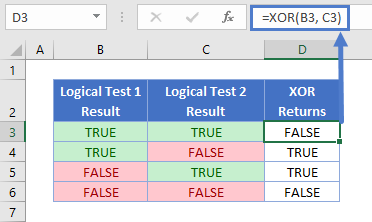XOR returns TRUE if an odd number of the arguments evaluate to TRUE, and FALSE if an even number of the arguments evaluate to TRUE.

Using XOR with Two Arguments

The most common usage of XOR by far, is when you only have two arguments in the function. This means that Excel will return TRUE if either one of the arguments is true, and FALSE if both, or none of the arguments is true. Here’s an example.

You’re the owner of a sports bar, and you want to give your staff a bonus if they sell over \$800 of food, and \$800 drinks in a given week. However, you’re feeling generous, so if they sell over \$800 in either food or drink, but not both, you’ll give them a half bonus.

Here are the takings for last week: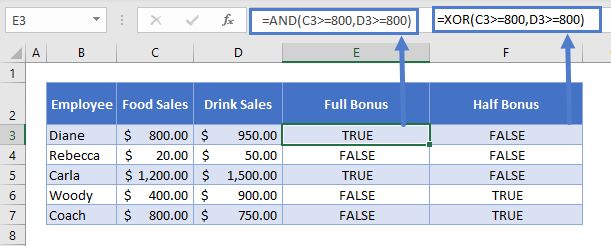In column E you’ve calculated the full bonus with Excel’s AND Function <<link>>:

This returns TRUE if both food sales and drink sales are over \$800.

And you can calculate the half bonus with the XOR function:

Note that the arguments in both functions are exactly the same, but XOR evaluates these arguments very differently to AND.

Using XOR with More than Two Arguments

Although using more than two arguments is less common in Excel, you can define up to 255 arguments in XOR.

However many you define, XOR will return an TRUE if an odd number evaluate to TRUE, and FALSE when an even number of them evaluate to TRUE.

See below: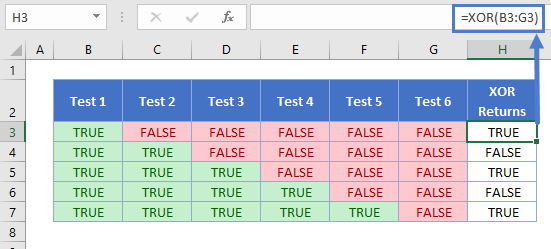To save time, you can supply a cell range here rather than separating the cell references with commas.

Comparing Text with XOR

Note that text comparisons are not case-sensitive. So the following formulas all produce the same result: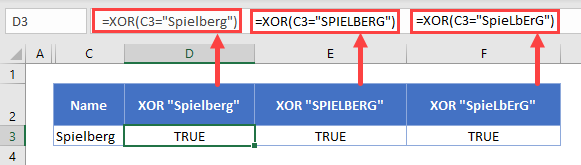Also, XOR does not support wildcards. So if you used the following formula: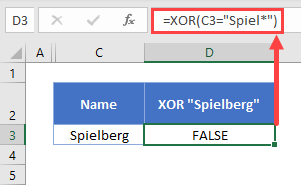XOR will look for an exact match against the text string “Spiel*”.

Comparing Numbers

You have a range of comparison operators at your disposal when comparing numbers. These are: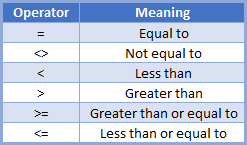If an expression in the XOR Function evaluates to a non-zero number, XOR will interpret that logical test as TRUE. If an expression is or evaluates to 0, XOR evaluates this to false. This is shown below: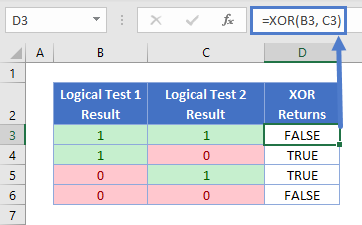Using XOR with IF

XOR is often used as part of a logical test in an IF statement. Essentially, IF enables you to customize the return value of the XOR test. So instead of just saying “TRUE” or “FALSE”, you can return anything – text, numbers, or even another formula.

Going back to our sports bar bonus example from earlier, you could use it like this: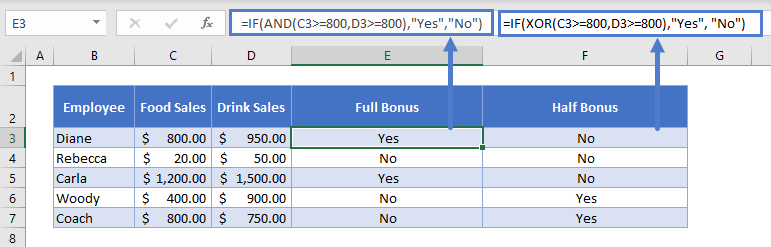IF first evaluates the XOR function. If it evaluates to TRUE, it then returns “Yes”. If it evaluates to FALSE, it returns “No”.

I’ve also added an IF statement to the “Full Bonus” formula in column E, which used the AND function rather than the XOR.

Combining IF with logical functions in this way can help make our data a little friendlier to the human eye.

Read more on the main page on the Excel IF Function <<link>>.

The XOR Function works exactly the same in Google Sheets as in Excel: